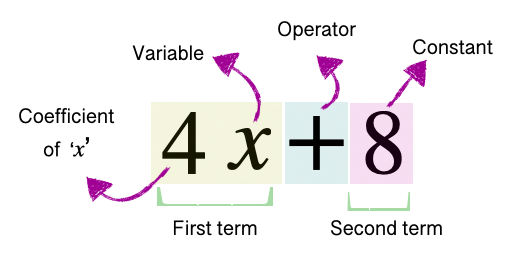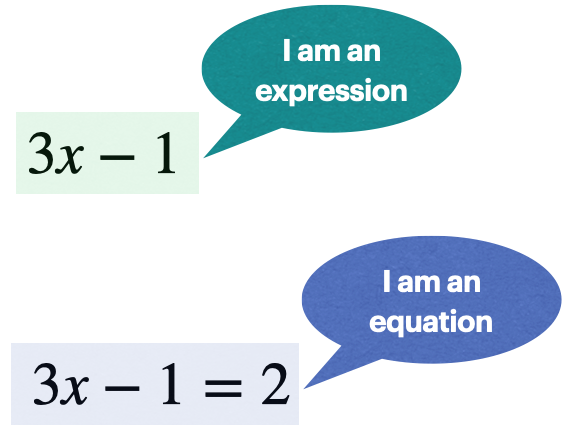# 7th Grade - Expressions and Equations

## Introduction

• An expression is a mathematical statement made up of numbers, variables, and arithmetic operators (such as addition and subtraction).
• Consider the example shown below to understand the different components of an expression.• Notice that an expression does not contain an equal sign or inequality symbol.
• The expressions that contain a variable are called algebraic expressions.
• The terms with the same variable(s) raised to the same exponent are called "like terms."
• Expressions and equations are not the same.
• An equation consists of two expressions separated by an equal sign.
• For example, $4x+8=2x-1$ is an equation.

## Difference between Expressions and Equations

• The main difference between an expression and an equation is that expressions don't equate to anything, whereas an equation does.
• Expressions are used to create equations.## Simplifying Expressions

• An algebraic expression is simplified in order to get a condensed equivalent algebraic expression.
• In order to simplify an algebraic expression, perform the following steps as applicable:
• Express the given expression in expanded form, if possible.
• Identify like terms.
• Combine like terms to get a simplified expression.
• Note: Obey the order of operation when combining like terms.

## Solving Equations

• In order to solve an equation, perform the following steps as applicable:
• Remove parenthesis
• Combine like terms
• Apply distributive property
• Solve using the division principle
• Solve using the multiplication principle
• Solve using the addition principle
• Solve using the subtraction principle

## Solved Examples

Question 1: Simplify the expression: $2x+10-\left(-x\right)+2$

Solution: $2x+10-\left(-x\right)+2$

Remove parenthesis:

$=2x+10+x+2$

Combining like terms:

$=3x+12$

Question 2: Solve the equation: $4x-3=2x+1$.

Solution: $4x-3=2x+1$

Subtraction principle:

$4x-3-2x=2x+1-2x$

$2x-3=1$

$2x-3+3=1+3$

$2x=4$

Division principle:

$\frac{2}{2}x=\frac{4}{2}$

$x=2$

Question 3: Solve the equation: $2\left(1-3x\right)+13=3\left(x-1\right)$.

Solution: $2\left(1-3x\right)+13=3\left(x-1\right)$

Remove parenthesis:

$2-6x+13=3x-3$

Subtraction principle:

$2-6x+13-3x=3x-3-3x$

$15-9x=-3$

Subtraction principle:

$15-9x-15=-3-15$

$-9x=-18$

Division principle:

$\frac{-9}{-9}x=\frac{-18}{-9}$

$x=2$

## Cheat Sheet

• To simplify an expression, we first express it in expanded form (get rid of parenthesis), identify and combine the like terms to get an expression in a compact form.
• To solve an equation, perform the following steps as applicable:
• Remove parenthesis
• Combine like terms
• Apply distributive property
• Solve using the division principle
• Solve using the multiplication principle
• Solve using the addition principle
• Solve using the subtraction principle

## Blunder Areas

• Remember that addition and subtraction operations can only be performed on like terms.